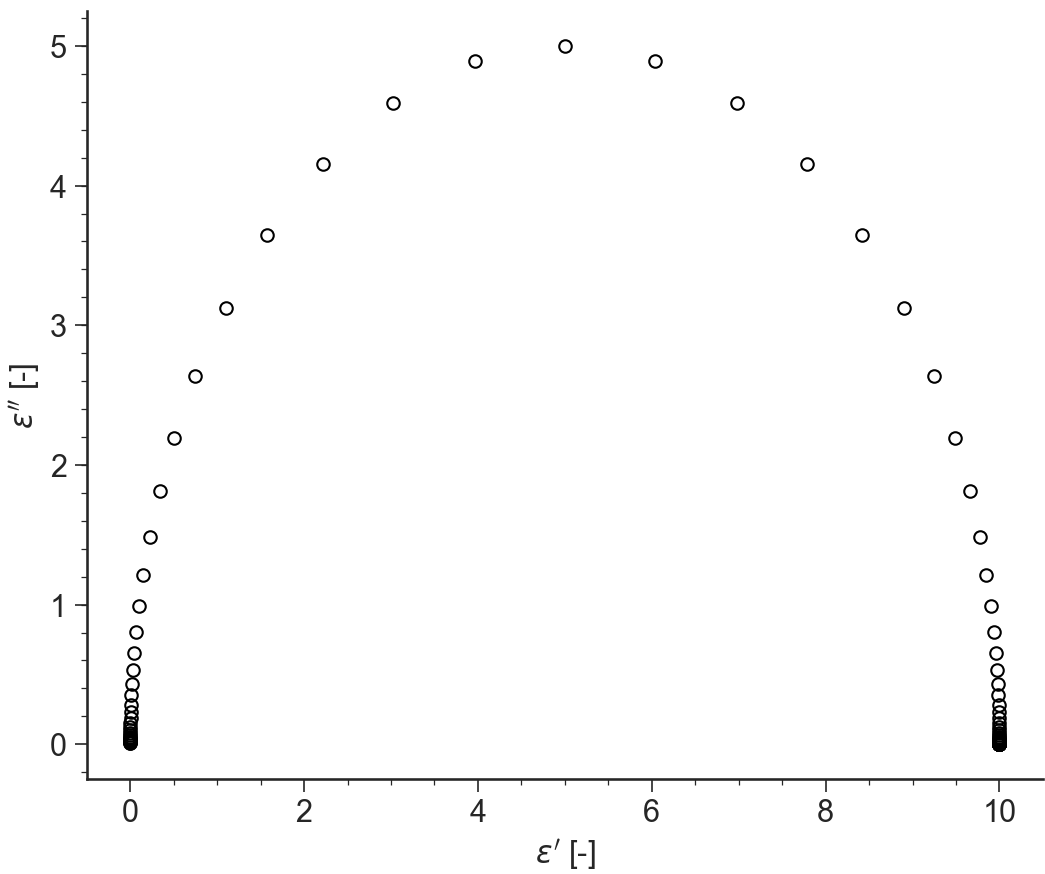# Dielectric: General Description¶

## Purpose¶

Application to Analyze Dielectric Spectroscopy Data

## Data Files¶

• The first line of the file should contain the sample parameters separated by semi-colons (;). It may contain any number of parameters which will be read and saved as file-parameter in RepTate.

• Then the data columns should appear, separated by spaces or tabs.

### .tts extension¶

Warning

NEEDS TO BE DONE

Text files with .dls extension should be organised as follows:

• .dls files should contaion at least the parameter values for the:

1. sample molar mass Mw,

2. temperature T.

• 3 columns separated by spaces or tabs containing respectively:

1. frequency, $$\omega$$,

2. elastic modulus, $$G'$$,

3. loss modulus $$G''$$.

Other columns will be ignored. A correct .tts file looks like:

T=-35;CTg=14.65;dx12=0;isof=true;Mw=13.5;chem=PI;PDI=1.04;
1.90165521264016E+0000      7.38023647054321E+0001      1.35152457625702E+0004     -2.99910000000000E+0001
3.01392554124040E+0000      1.99063258930248E+0002      2.14834778959042E+0004     -2.99900000000000E+0001
4.51700049635957E+0000      3.72861375546198E+0002      3.17756716623334E+0004     -3.99960000000000E+0001
...                         ...                         ...                        ...


## Views¶

### log(e’,e’’(w))¶

BaseApplicationDielectric.viewLogE1E2()[source]

Log or the relative permittivity and Dielectric Loss $$\epsilon'(\omega), \epsilon''(\omega)$$ vs logarithm of the frequency $$\omega$$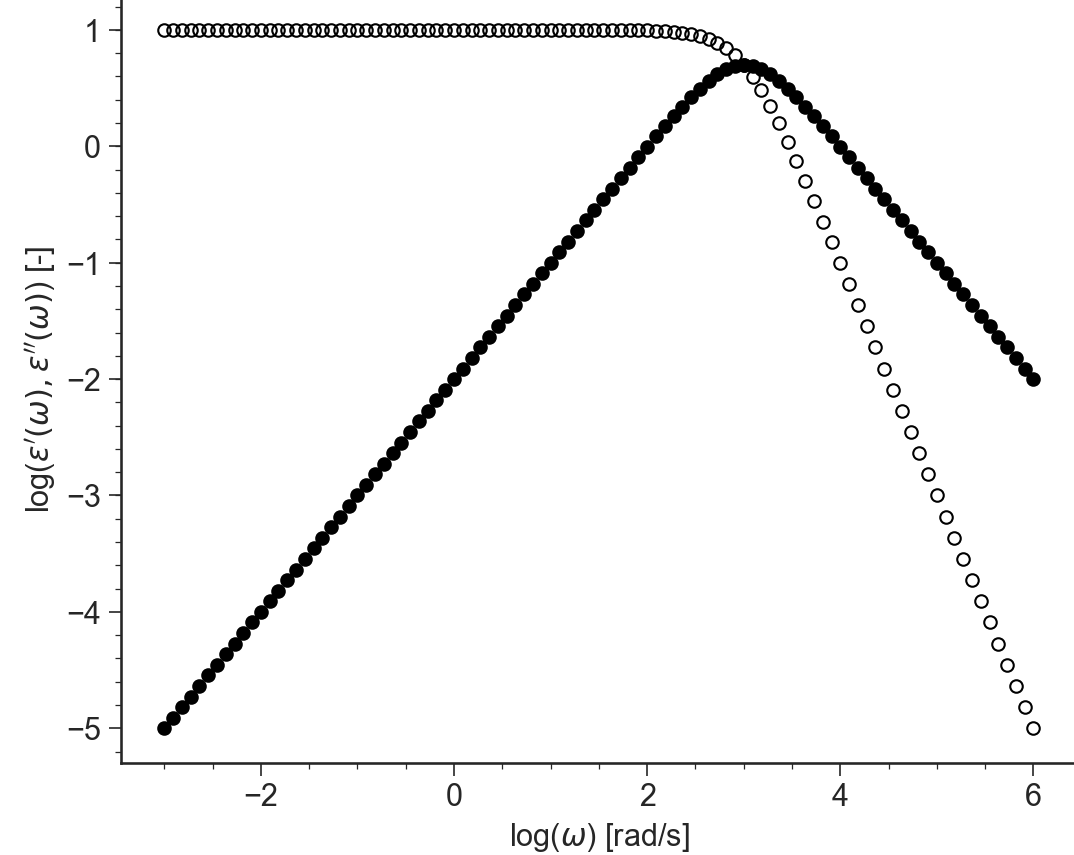### semilog(e’,e’’(w))¶

BaseApplicationDielectric.viewSemiLogE1E2()[source]

Semilog plot: Relative permittivity and Dielectric Loss $$\epsilon'(\omega), \epsilon''(\omega)$$ vs logarithm of frequency $$\omega$$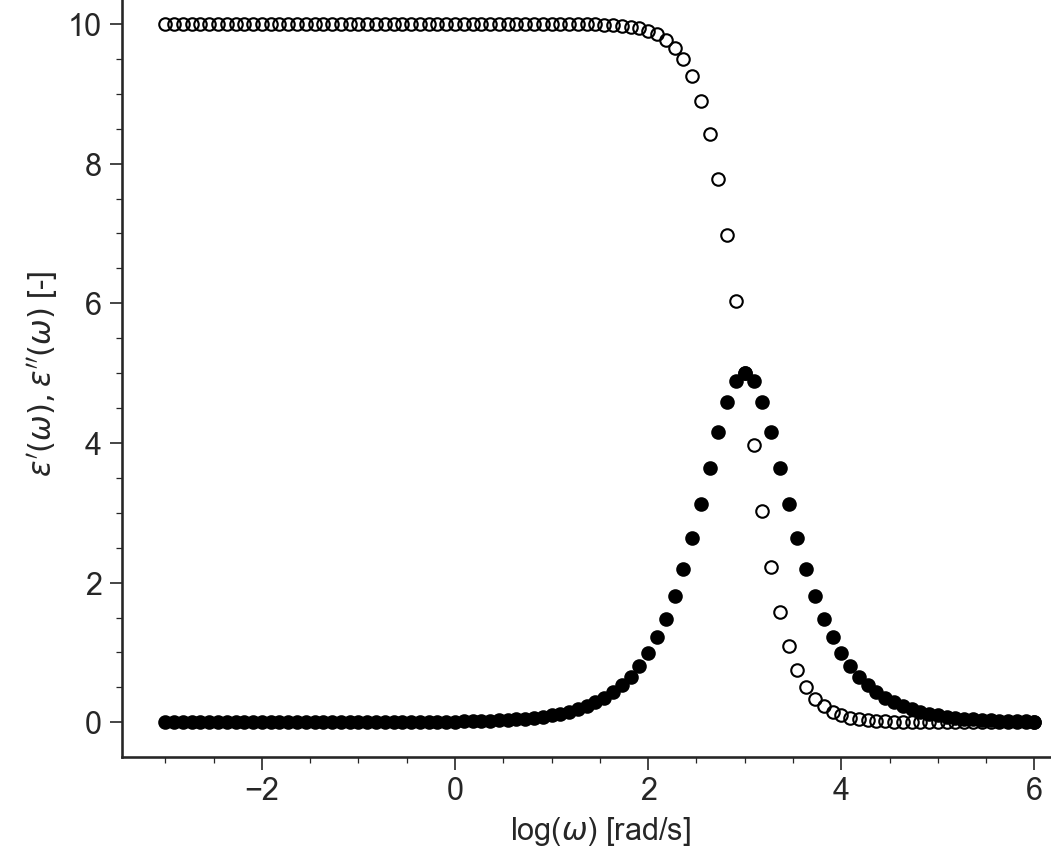### e’(w)”, “e’’(w)¶

BaseApplicationDielectric.viewE1E2()[source]

Relative permittivity and Dielectric Loss $$\epsilon'(\omega), \epsilon''(\omega)$$ vs frequency $$\omega$$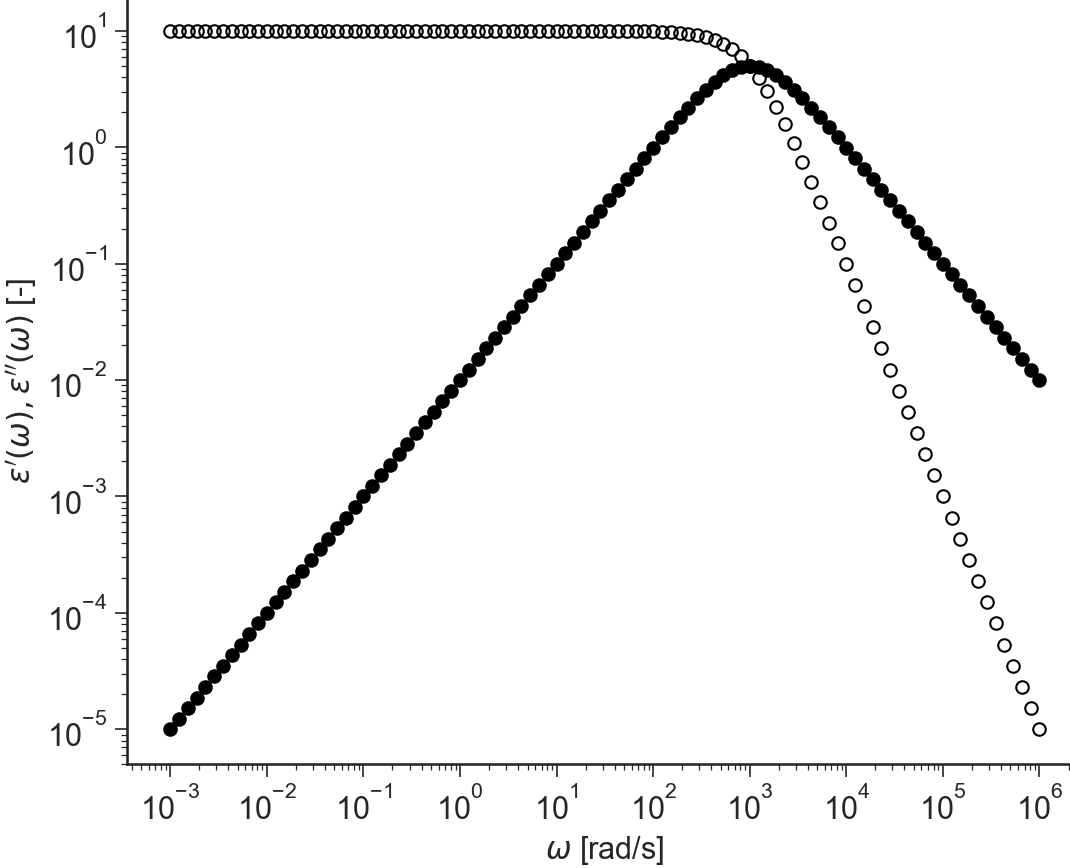### log(e’)¶

BaseApplicationDielectric.viewLogE1()[source]

Log or the relative permittivity $$\epsilon'(\omega)$$ vs logarithm of the frequency $$\omega$$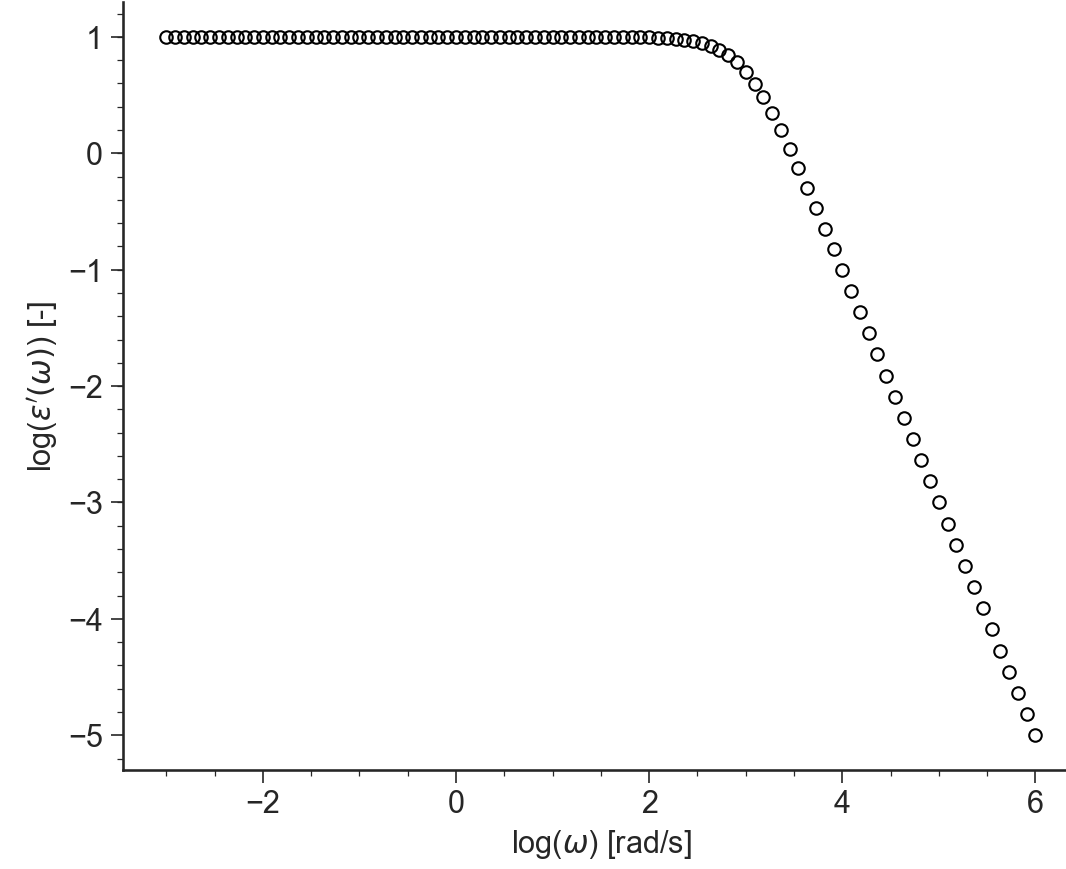### semilog(e’)¶

BaseApplicationDielectric.viewSemiLogE1()[source]

Semilog plot: Relative permittivity $$\epsilon'(\omega)$$ vs logarithm of the frequency $$\omega$$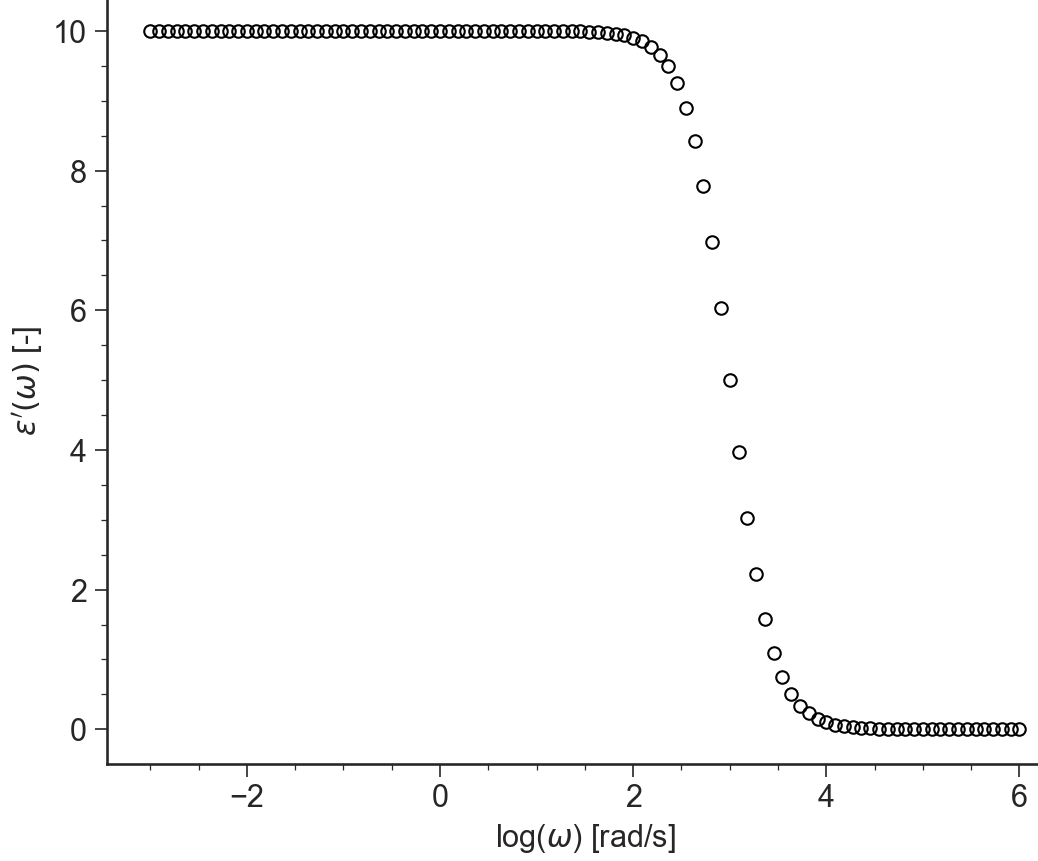### e’¶

BaseApplicationDielectric.viewE1()[source]

Relative permittivity $$\epsilon'(\omega)$$ vs frequency $$\omega$$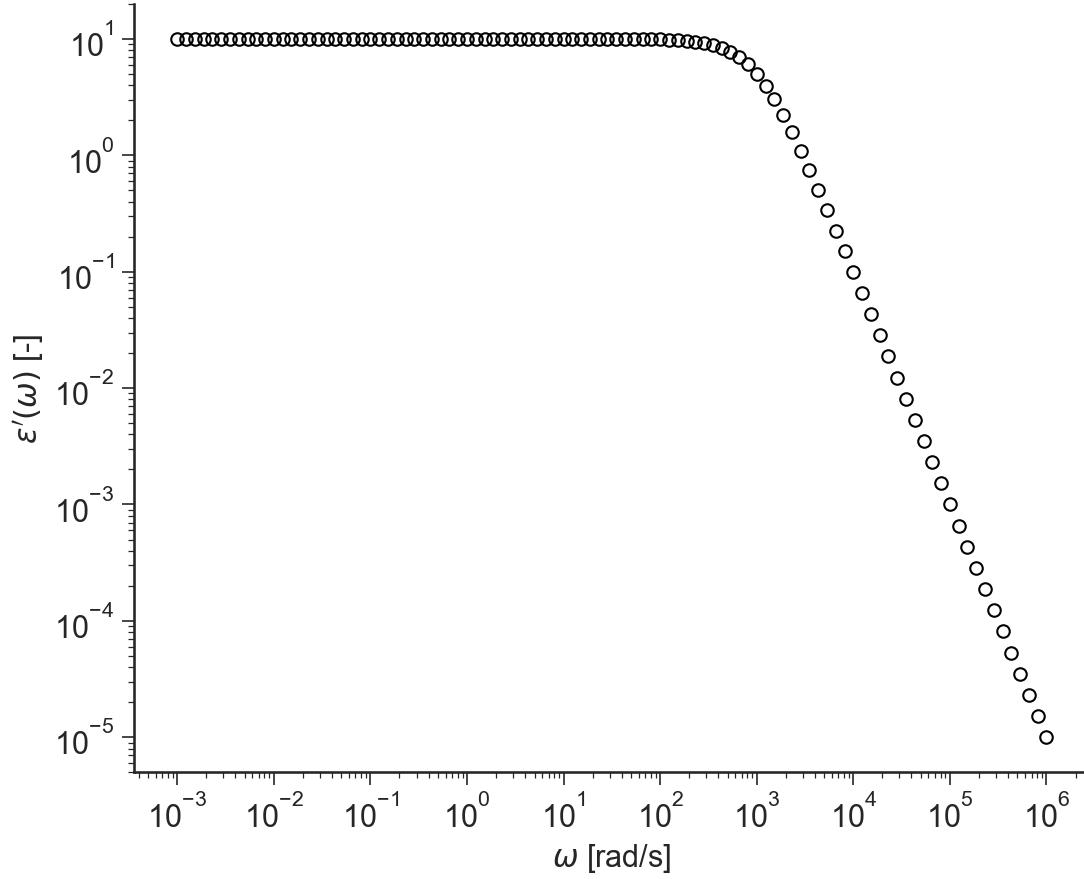### log(e’’)¶

BaseApplicationDielectric.viewLogE2()[source]

Log or the Dielectric Loss $$\epsilon''(\omega)$$ vs logarithm of the frequency $$\omega$$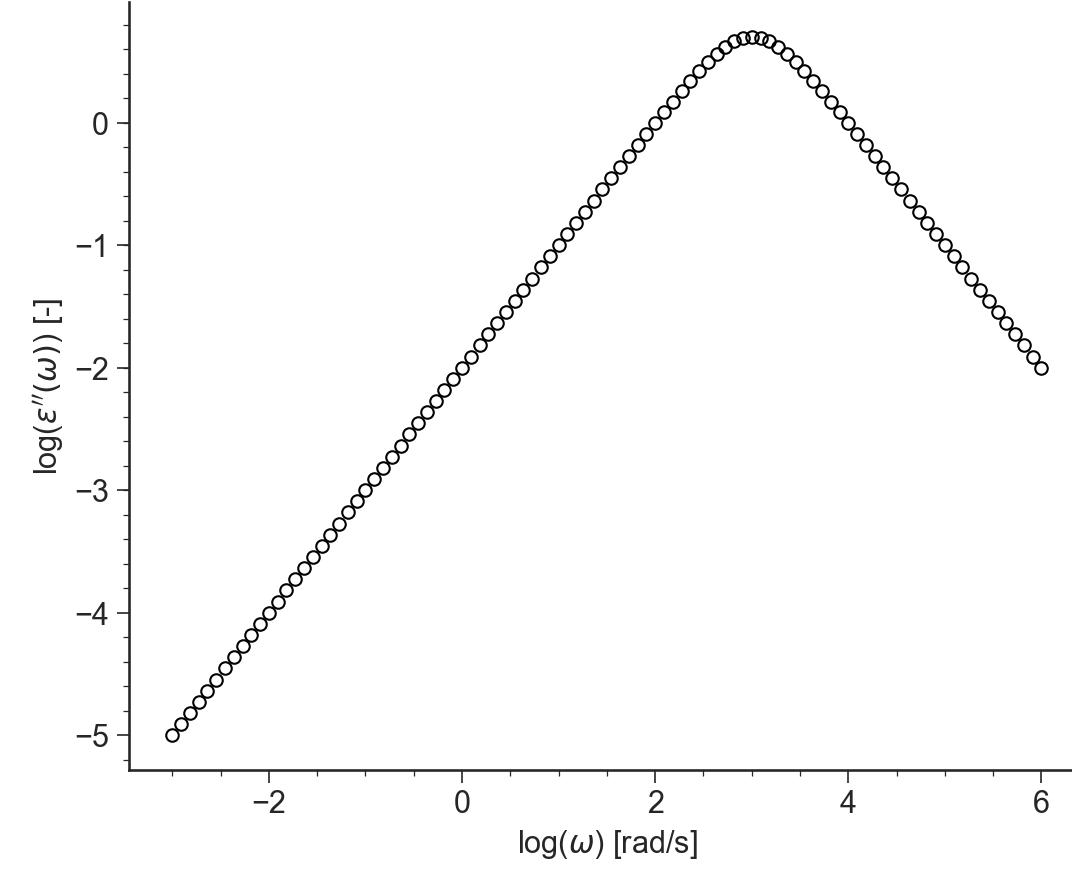### semilog(e’’)¶

BaseApplicationDielectric.viewSemiLogE2()[source]

Semilog plot: Dielectric Loss $$\epsilon''(\omega)$$ vs logarithm of the frequency $$\omega$$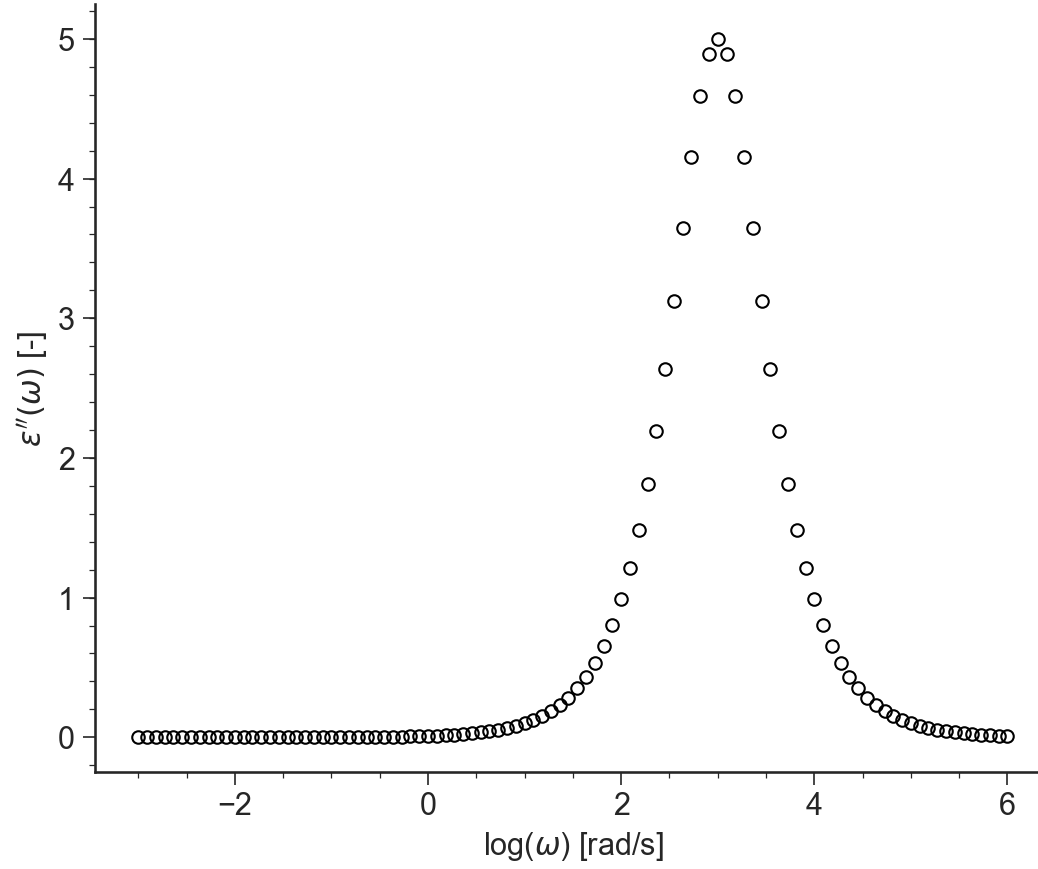### e’’¶

BaseApplicationDielectric.viewE2()[source]

Dielectric Loss $$\epsilon''(\omega)$$ vs frequency $$\omega$$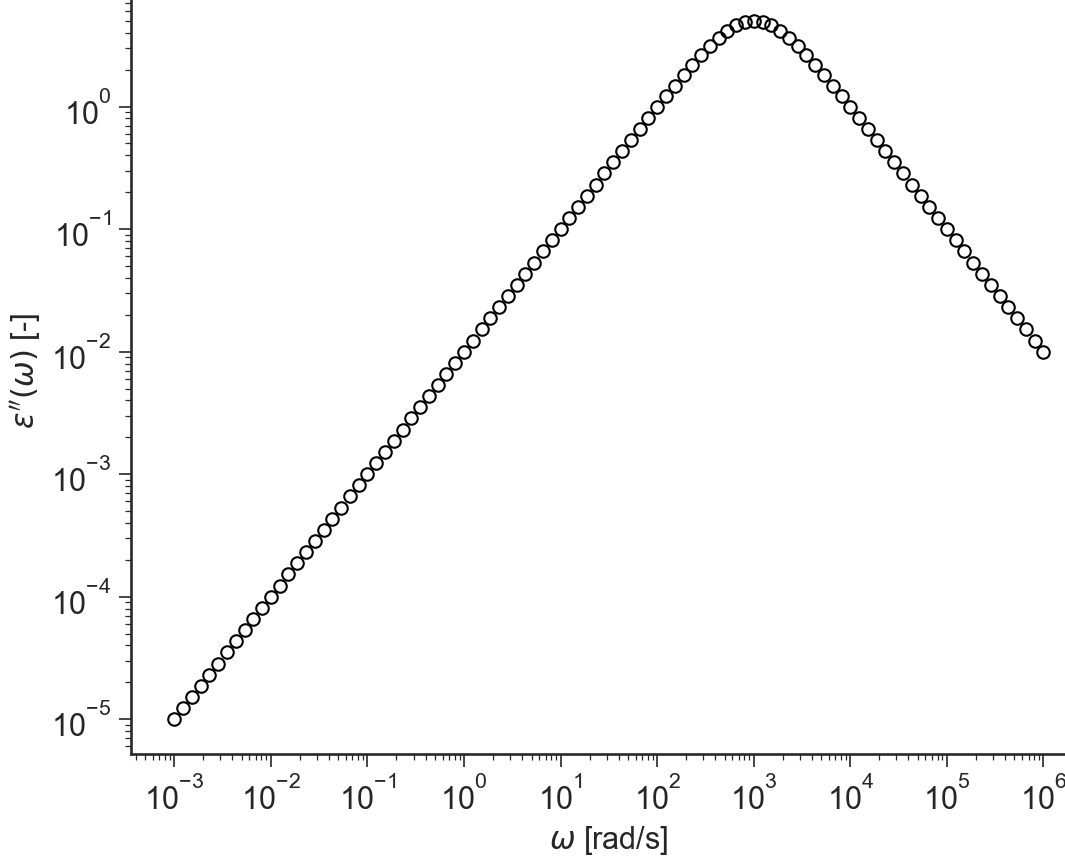### Cole-Cole¶

BaseApplicationDielectric.viewColeCole()[source]

Cole-Cole plot: Dielectric Loss $$\epsilon''(\omega)$$ vs relative permittivity $$\epsilon'(\omega)$$